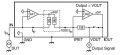# Two-stage Transimpedance Amplifier circuit question.

#### Robert Liang

Joined Dec 10, 2021
1Hi,

The image above is copied from a thesis for extremely small current measurements. The circuit provides a high I-V gain of 1G.
From one perspective, this circuit uses a two-stage trans-impedance amplifier topology, which the first providing a 1000-fold current gain, and the second performing a 1Mohm trans-impedance gain.

The question is from a different perspective, if I consider the 3G resistor as the first gain stage(trans-impedance), the 3M and 1M resistor as the second stage(invert voltage dumping amplifier), then why do I need this two-stage topology？（ Since the first 3G stage is large enough）

------
I guess this is because the output stage of OA1 is a current source(realized by discrete transistors), and the second perspective above is wrong? But I'm not sure.

Question by the way, what are the benefits of a two-stage amplifier versus a single-stage one?